# ∈-NFA of L = (a* + b*)

• Last Updated : 23 Nov, 2020

Prerequisite – Introduction of Finite Automata

Non-Deterministic Finite Automata and ∈-Non-Deterministic Finite Automata are almost the same except for their transition function and there are a few special rules for the construction of ∈-NFA.

In ∈-NFA following are the states as follows.

```∈-NFA is defined in 5 tuple representation {Q, q0, Σ, δ, F} where
Q is the set of all states,
q0 is the initial state,
Σ is the set of input symbols,
δ is the transition function which is δ:Q × (Σ∪∈)->2Q and
F is the set of final states.
```

Rules for constructing ∈-NFA :

Simple rules for construction of ∈-NFA as follows.

```∈-NFA for a+ :
```

This structure is for a+ which means there must be at least one ‘a’ in the expression. It is preceded by epsilon and also succeeded by one. There is epsilon feedback from state q2 to q1 so that there can be more than one ‘a’ in the expression.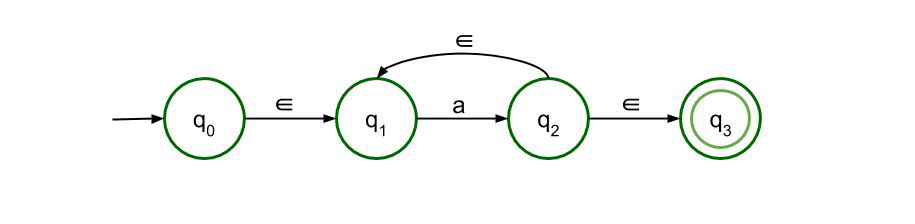```∈-NFA for a* :
```

This structure is for a* which means there can be any number of ‘a’ in the expression, even 0. The previous structure is just modified a bit to validate the null input symbol so, that expression is also valid.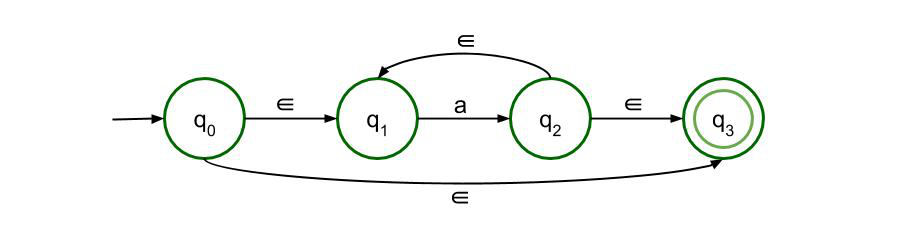```∈-NFA for a+b :
```

This structure accepts either a or b as input. So there are two paths, both of which lead to the final state.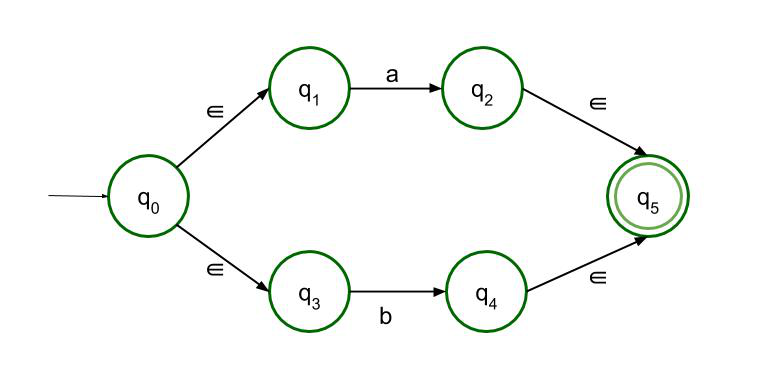```∈-NFA for ab :
```

For concatenation, a must be followed by b. Only then it can reach the final state. Both structures are allowed here but as it is ∈-NFA so the second structure is recommended.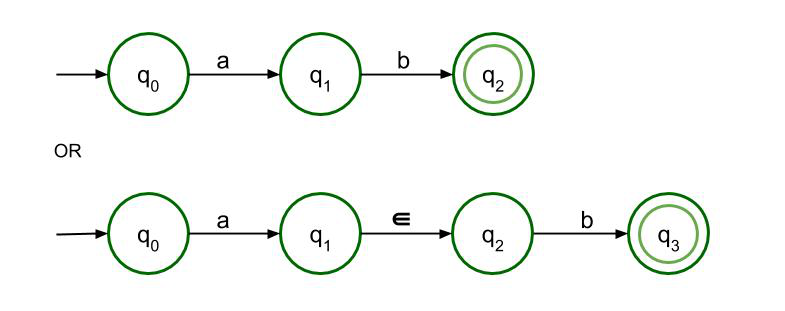```∈-NFA for L = (a* + b*) :
```

Following the above-mentioned rules, ∈-NFA of Regular Language L =a* + b* is to be constructed. The above language can be broken into two parts. The first part is a* and the second part is b* which can be constructed exactly like the first part. As there is a ‘+’ sign connecting the two parts, so there will be two paths, both of which will be leading to the final state.

The first part is a*, so it will be drawn exactly like the second rule. Again, using the second rule and replacing a with b, we get the second part, b*.

Final ∈-NFA :

The Final ∈-NFA will be :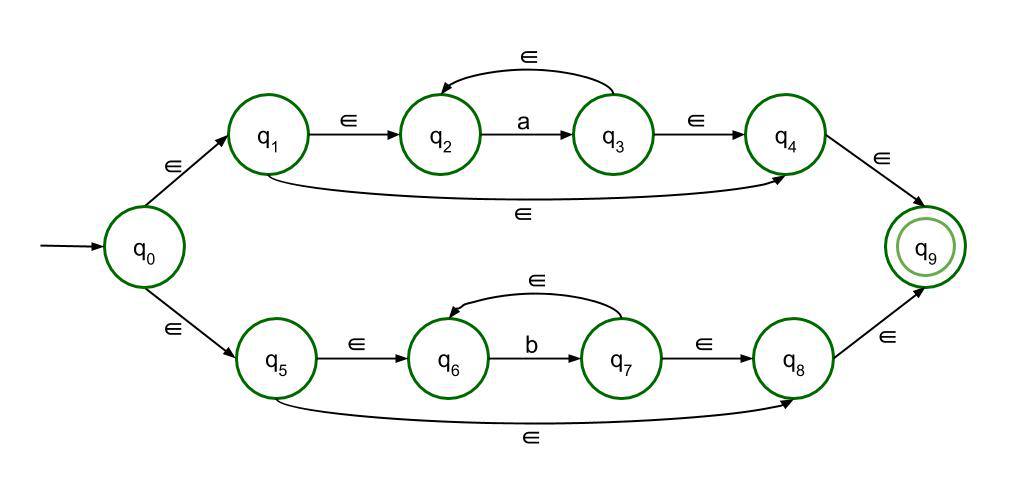My Personal Notes arrow_drop_up
Recommended Articles
Page :